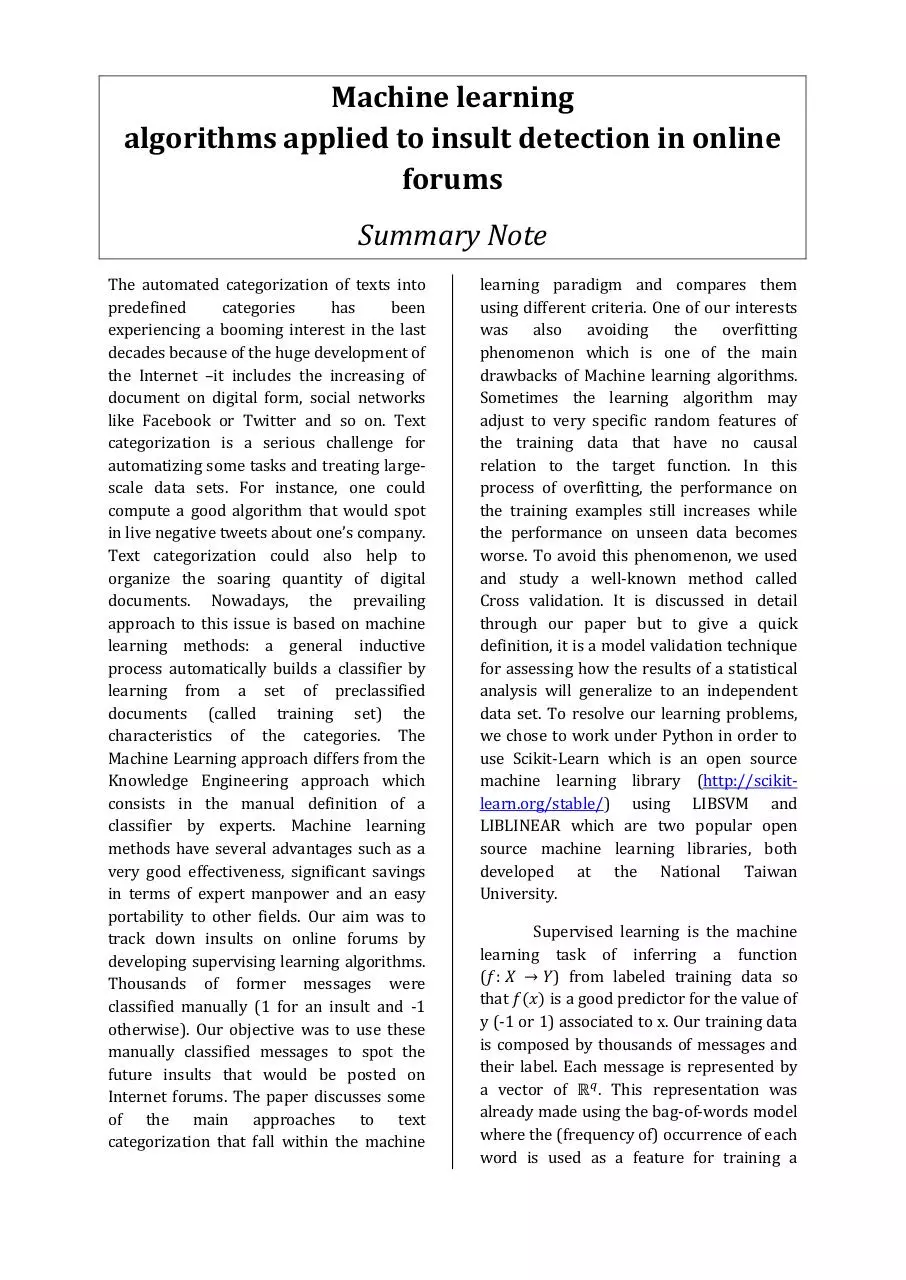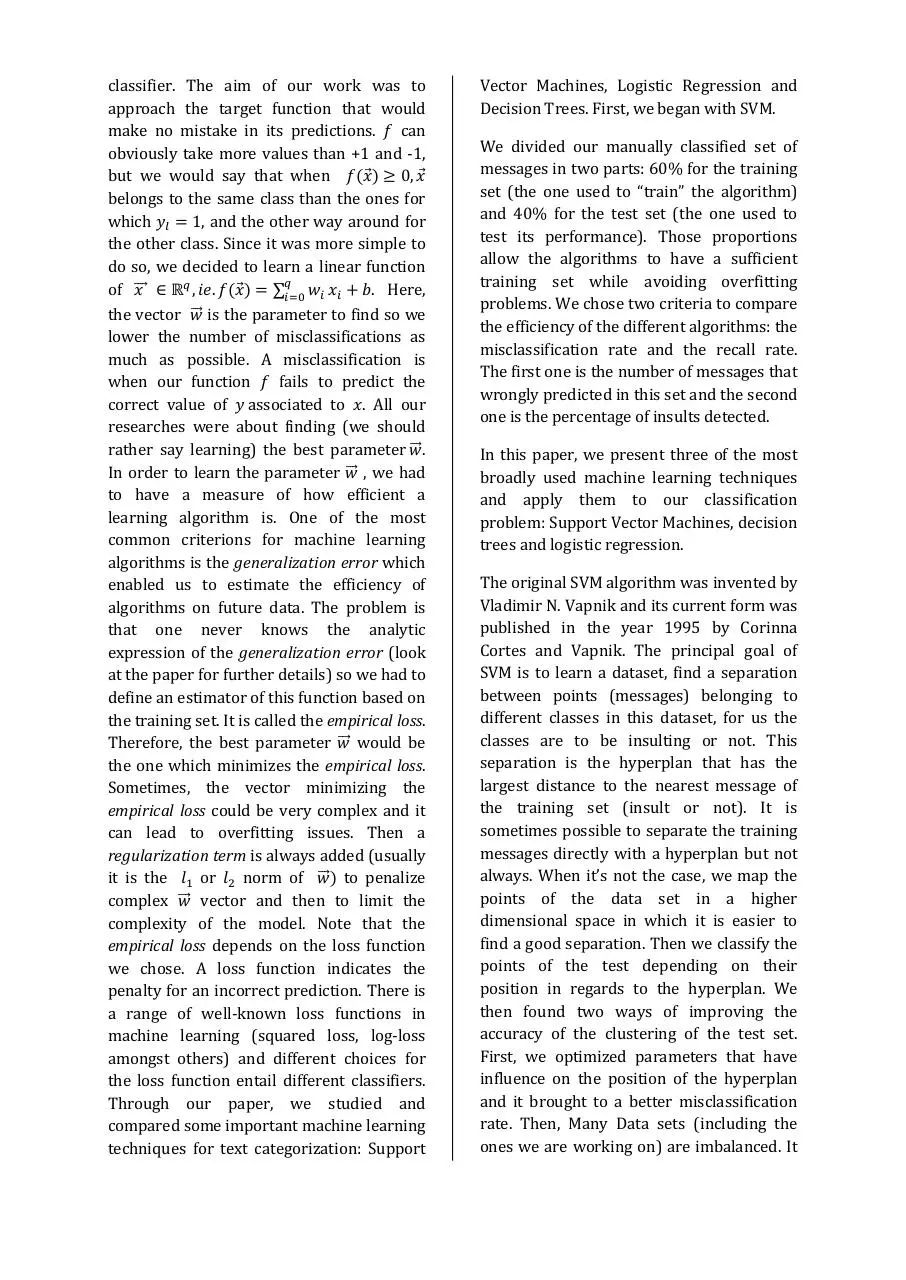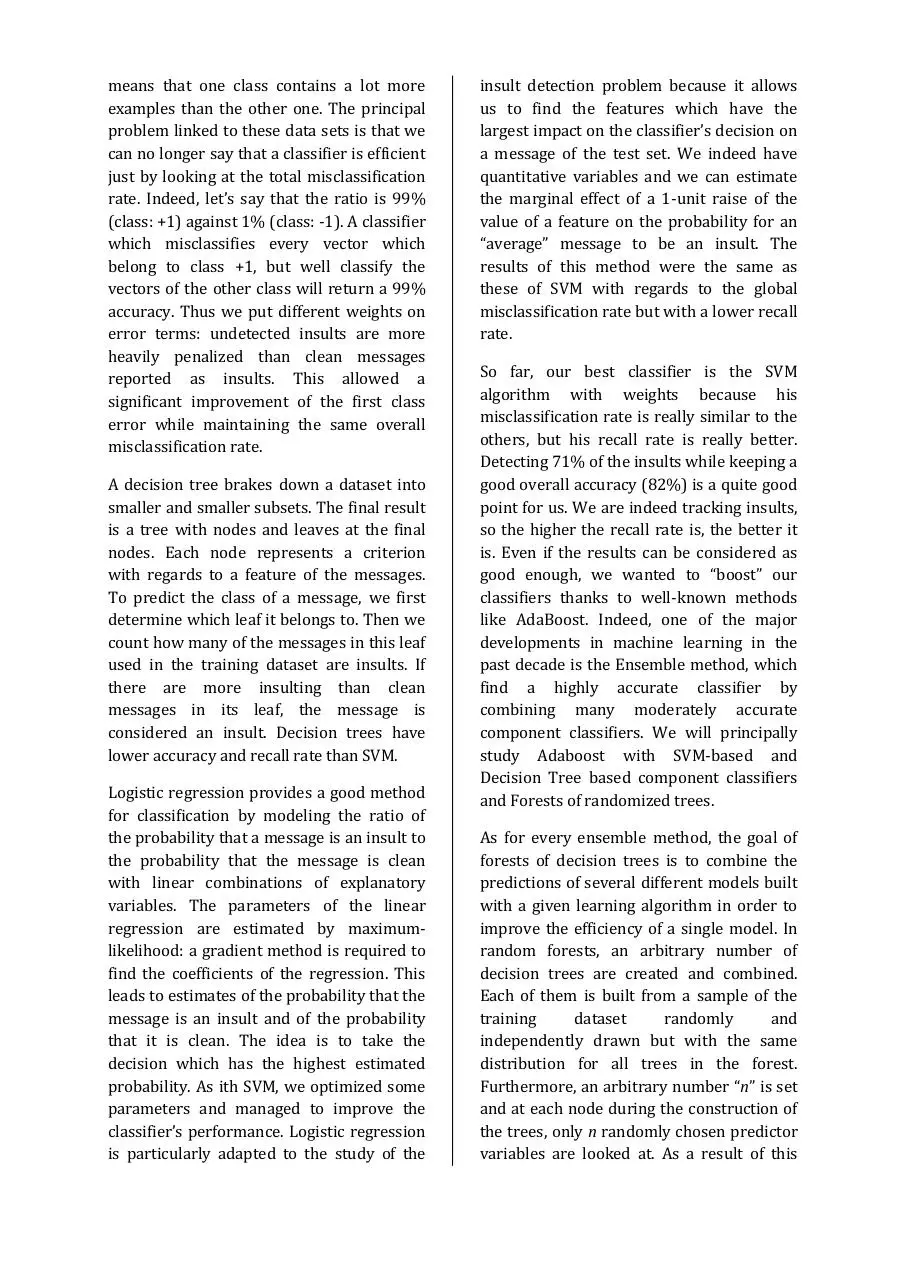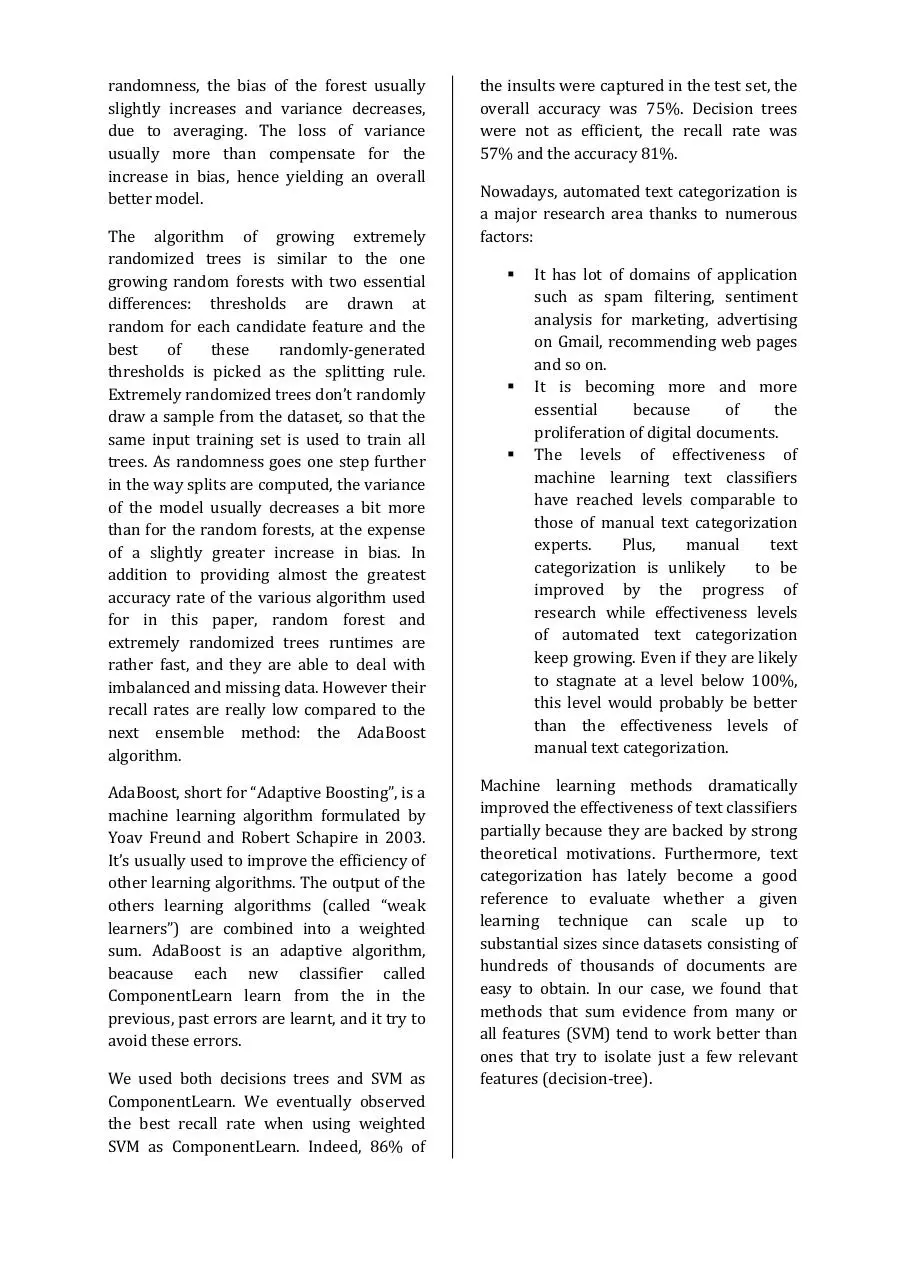# Summary Note Dehar Hamdaoui Lecocq Sitbon .pdf

### File information

Author: Nasir Jones

This PDF 1.5 document has been generated by MicrosoftÂ® Word 2010, and has been sent on pdf-archive.com on 08/05/2014 at 17:46, from IP address 46.193.x.x. The current document download page has been viewed 415 times.
File size: 523.52 KB (4 pages).
Privacy: public file

### Document preview

Machine learning
algorithms applied to insult detection in online
forums
Summary Note
The automated categorization of texts into
predefined
categories
has
been
experiencing a booming interest in the last
decades because of the huge development of
the Internet –it includes the increasing of
document on digital form, social networks
categorization is a serious challenge for
automatizing some tasks and treating largescale data sets. For instance, one could
compute a good algorithm that would spot
in live negative tweets about one’s company.
Text categorization could also help to
organize the soaring quantity of digital
approach to this issue is based on machine
learning methods: a general inductive
process automatically builds a classifier by
learning from a set of preclassified
documents (called training set) the
characteristics of the categories. The
Machine Learning approach differs from the
Knowledge Engineering approach which
consists in the manual definition of a
classifier by experts. Machine learning
methods have several advantages such as a
very good effectiveness, significant savings
in terms of expert manpower and an easy
portability to other fields. Our aim was to
track down insults on online forums by
developing supervising learning algorithms.
Thousands of former messages were
classified manually (1 for an insult and -1
otherwise). Our objective was to use these
manually classified messages to spot the
future insults that would be posted on
Internet forums. The paper discusses some
of the main approaches to text
categorization that fall within the machine

using different criteria. One of our interests
was
also
avoiding the overfitting
phenomenon which is one of the main
drawbacks of Machine learning algorithms.
Sometimes the learning algorithm may
adjust to very specific random features of
the training data that have no causal
relation to the target function. In this
process of overfitting, the performance on
the training examples still increases while
the performance on unseen data becomes
worse. To avoid this phenomenon, we used
and study a well-known method called
Cross validation. It is discussed in detail
through our paper but to give a quick
definition, it is a model validation technique
for assessing how the results of a statistical
analysis will generalize to an independent
data set. To resolve our learning problems,
we chose to work under Python in order to
use Scikit-Learn which is an open source
machine learning library (http://scikitlearn.org/stable/) using LIBSVM and
LIBLINEAR which are two popular open
source machine learning libraries, both
developed at the National Taiwan
University.
Supervised learning is the machine
learning task of inferring a function
(
) from labeled training data so
that
is a good predictor for the value of
y (-1 or 1) associated to x. Our training data
is composed by thousands of messages and
their label. Each message is represented by
a vector of
. This representation was
where the (frequency of) occurrence of each
word is used as a feature for training a

classifier. The aim of our work was to
approach the target function that would
make no mistake in its predictions. can
obviously take more values than +1 and -1,
but we would say that when

belongs to the same class than the ones for
which
, and the other way around for
the other class. Since it was more simple to
do so, we decided to learn a linear function

of ⃗⃗⃗⃗

. Here,
the vector ⃗⃗⃗ is the parameter to find so we
lower the number of misclassifications as
much as possible. A misclassification is
when our function
fails to predict the
correct value of associated to . All our
researches were about finding (we should
rather say learning) the best parameter ⃗⃗⃗.
In order to learn the parameter ⃗⃗⃗ , we had
to have a measure of how efficient a
learning algorithm is. One of the most
common criterions for machine learning
algorithms is the generalization error which
enabled us to estimate the efficiency of
algorithms on future data. The problem is
that one never knows the analytic
expression of the generalization error (look
at the paper for further details) so we had to
define an estimator of this function based on
the training set. It is called the empirical loss.
Therefore, the best parameter ⃗⃗⃗ would be
the one which minimizes the empirical loss.
Sometimes, the vector minimizing the
empirical loss could be very complex and it
can lead to overfitting issues. Then a
regularization term is always added (usually
it is the
or
norm of ⃗⃗⃗ to penalize
complex ⃗⃗⃗ vector and then to limit the
complexity of the model. Note that the
empirical loss depends on the loss function
we chose. A loss function indicates the
penalty for an incorrect prediction. There is
a range of well-known loss functions in
machine learning (squared loss, log-loss
amongst others) and different choices for
the loss function entail different classifiers.
Through our paper, we studied and
compared some important machine learning
techniques for text categorization: Support

Vector Machines, Logistic Regression and
Decision Trees. First, we began with SVM.
We divided our manually classified set of
messages in two parts: 60% for the training
set (the one used to “train” the algorithm)
and 40% for the test set (the one used to
test its performance). Those proportions
allow the algorithms to have a sufficient
training set while avoiding overfitting
problems. We chose two criteria to compare
the efficiency of the different algorithms: the
misclassification rate and the recall rate.
The first one is the number of messages that
wrongly predicted in this set and the second
one is the percentage of insults detected.
In this paper, we present three of the most
and apply them to our classification
problem: Support Vector Machines, decision
trees and logistic regression.
The original SVM algorithm was invented by
Vladimir N. Vapnik and its current form was
published in the year 1995 by Corinna
Cortes and Vapnik. The principal goal of
SVM is to learn a dataset, find a separation
between points (messages) belonging to
different classes in this dataset, for us the
classes are to be insulting or not. This
separation is the hyperplan that has the
largest distance to the nearest message of
the training set (insult or not). It is
sometimes possible to separate the training
messages directly with a hyperplan but not
always. When it’s not the case, we map the
points of the data set in a higher
dimensional space in which it is easier to
find a good separation. Then we classify the
points of the test depending on their
position in regards to the hyperplan. We
then found two ways of improving the
accuracy of the clustering of the test set.
First, we optimized parameters that have
influence on the position of the hyperplan
and it brought to a better misclassification
rate. Then, Many Data sets (including the
ones we are working on) are imbalanced. It

means that one class contains a lot more
examples than the other one. The principal
problem linked to these data sets is that we
can no longer say that a classifier is efficient
just by looking at the total misclassification
rate. Indeed, let’s say that the ratio is 99%
(class: +1) against 1% (class: -1). A classifier
which misclassifies every vector which
belong to class +1, but well classify the
vectors of the other class will return a 99%
accuracy. Thus we put different weights on
error terms: undetected insults are more
heavily penalized than clean messages
reported as insults. This allowed a
significant improvement of the first class
error while maintaining the same overall
misclassification rate.
A decision tree brakes down a dataset into
smaller and smaller subsets. The final result
is a tree with nodes and leaves at the final
nodes. Each node represents a criterion
with regards to a feature of the messages.
To predict the class of a message, we first
determine which leaf it belongs to. Then we
count how many of the messages in this leaf
used in the training dataset are insults. If
there are more insulting than clean
messages in its leaf, the message is
considered an insult. Decision trees have
lower accuracy and recall rate than SVM.
Logistic regression provides a good method
for classiﬁcation by modeling the ratio of
the probability that a message is an insult to
the probability that the message is clean
with linear combinations of explanatory
variables. The parameters of the linear
regression are estimated by maximumlikelihood: a gradient method is required to
find the coefficients of the regression. This
leads to estimates of the probability that the
message is an insult and of the probability
that it is clean. The idea is to take the
decision which has the highest estimated
probability. As ith SVM, we optimized some
parameters and managed to improve the
classifier’s performance. Logistic regression
is particularly adapted to the study of the

insult detection problem because it allows
us to find the features which have the
largest impact on the classifier’s decision on
a message of the test set. We indeed have
quantitative variables and we can estimate
the marginal effect of a 1-unit raise of the
value of a feature on the probability for an
“average” message to be an insult. The
results of this method were the same as
these of SVM with regards to the global
misclassification rate but with a lower recall
rate.
So far, our best classifier is the SVM
algorithm with weights because his
misclassification rate is really similar to the
others, but his recall rate is really better.
Detecting 71% of the insults while keeping a
good overall accuracy (82%) is a quite good
point for us. We are indeed tracking insults,
so the higher the recall rate is, the better it
is. Even if the results can be considered as
good enough, we wanted to “boost” our
classifiers thanks to well-known methods
like AdaBoost. Indeed, one of the major
developments in machine learning in the
past decade is the Ensemble method, which
find a highly accurate classiﬁer by
combining many moderately accurate
component classiﬁers. We will principally
Decision Tree based component classifiers
and Forests of randomized trees.
As for every ensemble method, the goal of
forests of decision trees is to combine the
predictions of several different models built
with a given learning algorithm in order to
improve the efficiency of a single model. In
random forests, an arbitrary number of
decision trees are created and combined.
Each of them is built from a sample of the
training
dataset
randomly
and
independently drawn but with the same
distribution for all trees in the forest.
Furthermore, an arbitrary number “n” is set
and at each node during the construction of
the trees, only n randomly chosen predictor
variables are looked at. As a result of this

randomness, the bias of the forest usually
slightly increases and variance decreases,
due to averaging. The loss of variance
usually more than compensate for the
increase in bias, hence yielding an overall
better model.
The algorithm of growing extremely
randomized trees is similar to the one
growing random forests with two essential
differences: thresholds are drawn at
random for each candidate feature and the
best
of
these
randomly-generated
thresholds is picked as the splitting rule.
Extremely randomized trees don’t randomly
draw a sample from the dataset, so that the
same input training set is used to train all
trees. As randomness goes one step further
in the way splits are computed, the variance
of the model usually decreases a bit more
than for the random forests, at the expense
of a slightly greater increase in bias. In
addition to providing almost the greatest
accuracy rate of the various algorithm used
for in this paper, random forest and
extremely randomized trees runtimes are
rather fast, and they are able to deal with
imbalanced and missing data. However their
recall rates are really low compared to the
algorithm.
machine learning algorithm formulated by
Yoav Freund and Robert Schapire in 2003.
It’s usually used to improve the efficiency of
other learning algorithms. The output of the
others learning algorithms (called “weak
learners”) are combined into a weighted
beacause each new classifier called
ComponentLearn learn from the in the
previous, past errors are learnt, and it try to
avoid these errors.
We used both decisions trees and SVM as
ComponentLearn. We eventually observed
the best recall rate when using weighted
SVM as ComponentLearn. Indeed, 86% of

the insults were captured in the test set, the
overall accuracy was 75%. Decision trees
were not as efficient, the recall rate was
57% and the accuracy 81%.
a major research area thanks to numerous
factors:

It has lot of domains of application
such as spam filtering, sentiment
on Gmail, recommending web pages
and so on.
It is becoming more and more
essential
because
of
the
proliferation of digital documents.
The levels of effectiveness of
machine learning text classifiers
have reached levels comparable to
those of manual text categorization
experts.
Plus,
manual
text
categorization is unlikely
to be
improved by the progress of
research while effectiveness levels
of automated text categorization
keep growing. Even if they are likely
to stagnate at a level below 100%,
this level would probably be better
than the effectiveness levels of
manual text categorization.

Machine learning methods dramatically
improved the effectiveness of text classifiers
partially because they are backed by strong
theoretical motivations. Furthermore, text
categorization has lately become a good
reference to evaluate whether a given
learning technique can scale up to
substantial sizes since datasets consisting of
hundreds of thousands of documents are
easy to obtain. In our case, we found that
methods that sum evidence from many or
all features (SVM) tend to work better than
ones that try to isolate just a few relevant
features (decision-tree).Summary Note - Dehar Hamdaoui Lecocq Sitbon.pdf (PDF, 523.52 KB)

### Share on social networks

#### HTML Code

Copy the following HTML code to share your document on a Website or Blog